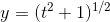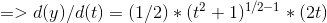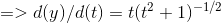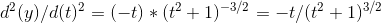# Problem in applying the Chain Rule

Gold Member

## Homework Statement

I am facing problem in applying the chain rule.
The question which I am trying to solve is,
" Find the second derivative of"

## Homework Equations## The Attempt at a Solution

So, differentiated it the first time,[BY CHAIN RULE]And now to find the second derivative I differentiated it once again,
so,=>But this is a wrong answer.
Please tell me where am I doing the mistake in applying the chain rule?
I will be thankful for help!

#### Attachments

•Delta2

fresh_42
Mentor
2021 Award

## Homework Statement

I am facing problem in applying the chain rule.
The question which I am trying to solve is,
" Find the second derivative of View attachment 227541 "

## Homework Equations

View attachment 227542

## The Attempt at a Solution

So, differentiated it the first time,
View attachment 227543 [BY CHAIN RULE]
View attachment 227544
And now to find the second derivative I differentiated it once again,
so,
View attachment 227545
=>View attachment 227546
But this is a wrong answer.
Please tell me where am I doing the mistake in applying the chain rule?
I will be thankful for help!
You differentiated ##\dfrac{d}{dx}(fg)## to ##\dfrac{d}{dx}(f)\cdot \dfrac{d}{dx}(g)## which it is not, and it is not the chain rule.
Do you know the chain rule? I would have expected this information under point 2. of the template.

Ray Vickson
Homework Helper
Dearly Missed

## Homework Statement

I am facing problem in applying the chain rule.
The question which I am trying to solve is,
" Find the second derivative of View attachment 227541 "

## Homework Equations

View attachment 227542

## The Attempt at a Solution

So, differentiated it the first time,
View attachment 227543 [BY CHAIN RULE]
View attachment 227544
And now to find the second derivative I differentiated it once again,
so,
View attachment 227545
=>View attachment 227546
But this is a wrong answer.
Please tell me where am I doing the mistake in applying the chain rule?
I will be thankful for help!

To get ##d^2y/dt^2## you need to apply the product rule to ##dy/dt##. That will produce two terms, not one, although you can then simplify it down to one term again.

Please do NOT attach images; it makes it difficult to cite results and sub-results. Since you already used some kind of package to format your formulas, why not type them in here directly, using LaTeX?

•Delta2
Delta2
Homework Helper
Gold Member
Your mistake is not in the application of the chain rule but you don't seem to apply correctly the product rule. You have find ##\frac{dy}{dt}## as product of ##t## and ##(t^2+1)^{-\frac{1}{2}}##. So to calculate the derivative of that product first apply correctly the product rule ##\frac{d(fg)}{dt}=\frac{df}{dt}g+f\frac{dg}{dt}## for ##f(t)=t## and ##g(t)=(t^2+1)^{-\frac{1}{2}}##and then apply the chain rule to calculate correctly ##\frac{dg}{dt}##.

•navneet9431